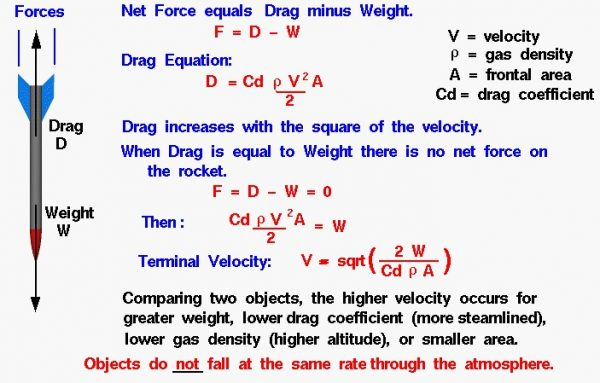# Terminal Velocity Interactive

Here is a JavaScript calculator which will solve the equations presented on this page:

Please note: the simulation below is best viewed on a desktop computer. It may take a few minutes for the simulation to load.## External Forces

An object which is falling through the atmosphere is subjected to two external forces. One force is the gravitational force, expressed as the weight of the object. The other force is the air resistance, or drag of the object. If the mass of an object remains constant, the motion of the object can be described by Newton’s second law of motion, force F equals mass m times acceleration a:

$$\LARGE F=ma$$

which can be solved for the acceleration of the object in terms of the net external force and the mass of the object:

$$\LARGE a=\frac{F}{m}$$

Weight and drag are forces which are vector quantities. The net external force F is then equal to the difference of the weight W and the drag D

$$\LARGE F=W-D$$

The acceleration of a falling object then becomes:

$$\LARGE a=\frac{W-D}{m}$$

The magnitude of the drag is given by the drag equation. Drag D depends on a drag coefficient Cd, the atmospheric density rho ($$\bf\rho$$), the square of the air velocity V, and some reference area A of the object.

$$\LARGE D=\mathit{Cd}\cdot\rho V^{2}\frac{A}{2}$$

## Drag

Drag increases with the square of the speed. So as an object falls, we quickly reach conditions where the drag becomes equal to the weight, if the weight is small. When drag is equal to weight, there is no net external force on the object and the vertical acceleration goes to zero. With no acceleration, the object falls at a constant velocity as described by Newton’s first law of motion. The constant vertical velocity is called the terminal velocity.

Using algebra, we can determine the value of the terminal velocity. At terminal velocity:

$$\LARGE D=W$$

$$\LARGE D=\mathit{Cd}\cdot\rho V^{2}\frac{A}{2}$$

Solving for the vertical velocity V, we obtain the equation:

$$\LARGE V=\sqrt{\frac{2W}{\mathit{Cd}\cdot\rho A}}$$

where $$\bf\sqrt{}$$ denotes the square root function.

## Terminal Velocity

The terminal velocity equation tells us that an object with a large cross-sectional area or a high drag coefficient falls slower than an object with a small area or low drag coefficient. A large flat plate falls slower than a small ball with the same weight. If we have two objects with the same area and drag coefficient, like two identically sized spheres, the lighter object falls slower. This seems to contradict the findings of Galileo that all free-falling objects fall at the same rate with equal air resistance. But Galileo’s principle only applies in a vacuum, where there is NO air resistance and drag is equal to zero.

## General Instructions

The chemistry of the atmosphere and the gravitational constant of a planet affects the terminal velocity. You select the planet using the choice button at the top left. The calculations can be performed in English (Imperial) or metric units. Specify the weight or mass of your object. You can choose to input either the weight on Earth, the local weight on the planet, or the mass of the object. Then you must specify the cross-sectional area and the drag coefficient. Finally, you must specify the atmospheric density. We have included models of the atmospheric density variation with altitude for Earth and Mars in the calculator. When you have the proper test conditions, press the red “Compute” button to calculate the terminal velocity.

Notice: In this calculator, you have to specify the drag coefficient. The value of the drag coefficient depends on the shape of the object and on compressibility effects in the flow. For airflow near and faster than the speed of sound, there is a large increase in the drag coefficient because of the formation of shock waves on the object. So be very careful when interpreting results with large terminal velocities. If your drag coefficient includes compressibility effects, then your answer is correct. If your drag coefficient was determined at low speeds, and the terminal velocity is very high, you are getting the wrong answer because your drag coefficient does not include compressibility effects.

Provide feedback